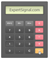Momentum Oscillator & Rate of Change

Usage: Identifying the Trend

◙ Trading: Detecting weak trends and likely reversal points

Standard Settings: 14, 21, 63, 125, 250 PeriodsIntroduction to the Momentum Oscillator

The Momentum oscillator measures the difference between last price and the price of T periods ago. The result is a series that oscillates around a horizontal equilibrium line (zero line), this line represents the level at which the price is unchanged from its reading T periods ago. Momentum is very closely related to the Rate of change (ROC), it will generate identical trading signals as the ROC (check below for more information about the Rate of Change).Calculating the Momentum Oscillator

This is Momentum Oscillator Formula:

Momentum = Closing Price – Closing Price (T periods ago)Trading with the Momentum Oscillator

The 'Momentum' is considered a simplistic indicator, these are some key points when trading with this oscillator:

-The 'Momentum' can be used for detecting trend weakness and potential points of trend reversal

-High indications (positive/negative) signify a strong trend

-Low indications signify the start or the end of a trend

-The 'Momentum can generate trade signals in both trending and ranging markets

-It is more reliable to trade the divergences between the slope of 'Momentum and the price chart slopePlatform Setup

□ GO TO → INDICATORS → OSCILLATORS → MOMENTUM

□ SETTINGS → 14 PERIODS

Rate of Change (ROC)

The ROC measures the change (%) between the current price and the price before a certain period, for example, before 21 days.

There are 250 trading days in a single year or 125 trading days per half year. There are trading 63 days per quarter and 21 trading days per month.

-In general, a trend reversal commences when the shortest timeframe spreads gradually to the other timeframes.

How ROC defines the Long-Term Trend -Conditions of Bulls and Bears

When ROC of 21 periods and ROC of 63 periods is above the 125 periods ROC and the 250 periods ROC then the long-term trend is considered bullish

When ROC of 21 periods and ROC of 63 periods is below the 125 periods ROC and the 250 periods ROC then the long-term trend is considered bearish

Calculating ROC

ROC = [(Close - Close 'n' periods ago)/ (Close 'n' periods ago)] * 100

Momentum Oscillator and Rate of Change (ROC)